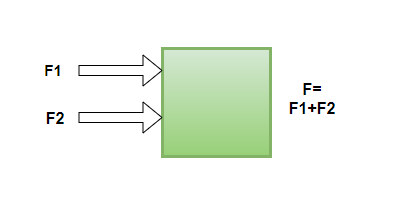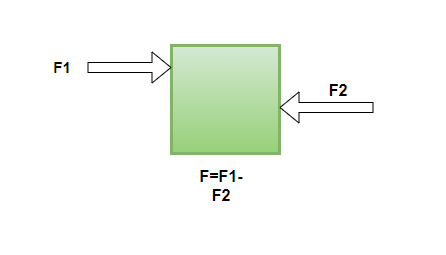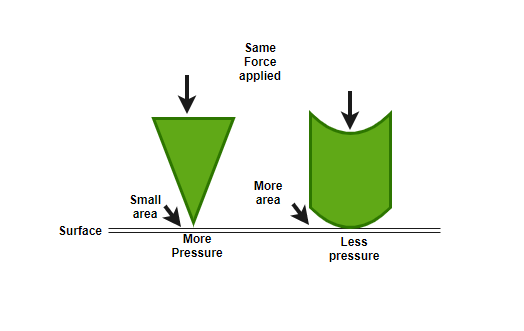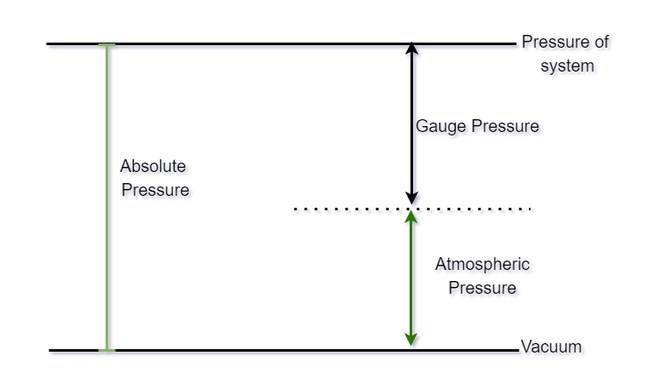GFG App
Open AppBrowser
Continue

Physics teaches that force is mass times acceleration, but if it is required to imagine what force is, and how does it affect a body? It is Simple. Imagine pushing or pulling an object, The push or pull of an object is applying force to the object, and if the object shows some displacement, there will be a finite value of Force. The force acting per unit area on a body is known as Pressure. Pressure and Force are closely related, the more the force on an object, the more will be the pressure on the object.

### Effect and Types of Force

When Force is applied to a body, It can start the object’s motion or can stop its motion, or can help in changing the shape or size of the object based on what type of force is applied. If a body experiences two forces, the forces may add up or may decrease the overall force. This is due to the reason that force is a vector quantity, which means, it has both magnitude and direction. Hence, the direction of force is also essential.

• If the forces applied to the object are in the same direction, the forces will add up and the overall force will be more.• If the forces applied to the object are in opposite direction, the forces will not add up and the overall force will be less.The formula for Force is given as, F = Ma.

The SI unit of Force is Newton (N)

There are many types of Forces that can be applied or naturally present in the universe. Contact Force is one type where the body should be in contact, for example, hitting a ball, or pushing a box. Muscular Force is the kind of Force where the muscles are in strain due to the applied force, For example, pushing any object applies force on the muscles. Friction Force is the type of force that is present between two surfaces, it resists the motion of the objects and always works in the direction opposite to motion.

Non-Contacting Force is the one where the bodies are not in contact, but the force is applied. Forces like Magnetic Force, Gravitational force are non-contacting forces. Electrostatic force is the one where two charges exert forces on each other Like charges repel and unlike charges attract each other. Gravitational force is exerted by two masses and the size of the mass acts most in producing the force, For example, Gravity is the Gravitational force exerted by Planet earth.

### Pressure

Pressure is defined as the Force per unit area. This directly means area affects the amount of pressure applied, if the area on which the force is applied is less, the pressure is less if the area is large on which force is applied.

The SI unit for pressure is N/m2. Also, Different types of Pressure have different units in standard form.

Formula for Pressure, P = F/A

Example of Pressure in real life:

• While cutting fruit or a vegetable, a sharp knife is used instead of a blunt one so that the pressure applied on the fruit is more and the fruits cut easily.
• The nails that are nailed on the wall are very pointy at the end in order to put more pressure on the wall.
• Porters put a round piece of cloth on their heads in order to increase the area and the pressure is less so that heavyweights can be applied.### Types of Pressure

There are different types of Pressure, and they have different units of pressure. For instance, atm is the unit used for atmospheric pressure. Let’s take a look at the different types of Pressure present,

Atmospheric Pressure:

Air is present above the sea level, and there are so many layers, the higher it goes, air starts to exert more and more pressure, and the pressure exerted by the air in the atmosphere is known as atmospheric pressure. The unit used for measuring the atmospheric pressure is atm.

The human body also has Pressure inside the body which compensates for the pressure present in the atmosphere, Therefore, Human body does not feel it. The atmospheric pressure varies due to the fluctuation in the atmosphere. The weight of air is responsible for the atmospheric pressure, and it is essential for the life on earth.

The instrument used to measure the atmospheric pressure is called Barometer. Barometer has Mercury filled, and it is vacuumed in the rest of the space. It measures Patm as pgh.

Patm = pgh

Where, p = Density of the air

g = acceleration due to gravity

h = height of the mercury.

Absolute Pressure:

Absolute Pressure is the pressure with respect to Zero pressure present, that is, with respect to no pressure present in the empty, free-space. No pressure is obtained in vacuum. Absolute pressure is denoted as Pabs

Differential Pressure:

Differential Pressure, as the name suggests, is the difference between the two values of pressure. The pressure obtained will be lesser than either of the pressures and unit is same for the differential pressure obtained.

Pd = P2 – P1

Gauge Pressure:

Gauge pressure is also known as Overpressure, and it is the recently invented pressure. The differential pressure obtained from atmospheric pressure and absolute pressure is known as overpressure or gauge pressure.

Below given figure shows the particular relation among Gauge Pressure, Absolute Pressure, Atmospheric pressure,Note: In order to measure Blood pressure, an inflatable cuff is placed on the forearm, Blood flow is detected and the corresponding pressures are measured in the mercury- filled manometer (Device used to measure pressure). The maximum blood pressure is called as Systolic Pressure. The minimum blood pressure is called as Diastolic pressure.

### Sample Problems

Question 1: The mass of an object is 50kgs and the object is accelerating, the acceleration is 2 m/sec2. Find the value of Force produced by the object.

Solution:

Given: Mass= 50kgs

Acceleration (a)= 2 m/sec2

Force = Mass × Acceleration

Force = 50 × 2

Force= 100N

Question 2: The Force Produced by a car is having a mass of 100kgs is equal to 300N. Find the acceleration of the car.

Solution:

Given : Mass= 100kgs

Force= 300N

Force = Mass × Acceleration

Acceleration = Force/Mass

Acceleration = 300/100

Acceleration (a)= 3 m/sec2

Question 3: What is the difference between Gauge pressure and differential pressure?

Differential pressure is the difference between the two pressure while gauge pressure or overpressure is defined as the difference between absolute pressure and atmospheric pressure. Therefore, we can conclude that gauge pressure can be differential pressure but not vice-versa.

Question 4: Why the straps provided by school bags are preferably made wider. What happens if the bag straps are less wide?

The bag straps are put on shoulders and based on how heavy the bag is, there is certain pressure exerted on the shoulder, if the straps are wider, the area covered would be more and hence, the pressure exerted on the shoulders would be less. However, if the straps are not wide enough, the area covered would be really less and the pressure exerted may injure the shoulders.

Question 5: An object is being pushed with a force of 50N and the base of the object is 10m2, What will be the pressure exerted by the object on the surface?

Solution:

Given: Force= 50N

Area= 10m2

Pressure= Force/area

Pressure(P) = 50/10

Pressure (P)= 5 N/m2

Question 6: An object exerted a pressure of 10N/m2, and the area for which the pressure is applied is 30m2. Find the amount of Force required?

Solution:

Given: Pressure= 10N/m2

Area= 30m2

Force= Pressure × Area

Force= 10 × 30

Force= 300N

My Personal Notes arrow_drop_up